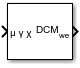# Wind Angles to Direction Cosine Matrix

Convert wind angles to direction cosine matrix

•Libraries:
Aerospace Blockset / Utilities / Axes Transformations

## Description

The Wind Angles to Direction Cosine Matrix block converts three wind rotation angles into a 3-by-3 direction cosine matrix (DCM). The DCM matrix performs the coordinate transformation of a vector in earth axes (ox0, oy0, oz0) into a vector in wind axes (ox3, oy3, oz3). For more information on the direction cosine matrix, see Algorithms.

This implementation generates a flight path angle that lies between ±90 degrees, and bank and heading angles that lie between ±180 degrees.

## Ports

### Input

expand all

Wind rotation angles, specified as a 3-by-1 vector, in radians.

Data Types: `double`

### Output

expand all

Direction cosine matrix, returned as a 3-by-3 matrix.

Data Types: `double`

## Algorithms

The DCM matrix performs the coordinate transformation of a vector in earth axes (ox0, oy0, oz0) into a vector in wind axes (ox3, oy3, oz3). The order of the axis rotations required to bring this about is:

1. A rotation about oz0 through the heading angle (χ) to axes (ox1, oy1, oz1)

2. A rotation about oy1 through the flight path angle (γ) to axes (ox2, oy2, oz2)

3. A rotation about ox2 through the bank angle (μ) to axes (ox3, oy3, oz3)

`$\begin{array}{l}\left[\begin{array}{c}o{x}_{3}\\ o{y}_{3}\\ o{z}_{3}\end{array}\right]=DC{M}_{we}\left[\begin{array}{c}o{x}_{0}\\ o{y}_{0}\\ o{z}_{0}\end{array}\right]\\ \\ \left[\begin{array}{c}o{x}_{3}\\ o{y}_{3}\\ o{z}_{3}\end{array}\right]=\left[\begin{array}{ccc}1& 0& 0\\ 0& \mathrm{cos}\mu & \mathrm{sin}\mu \\ 0& -\mathrm{sin}\mu & \mathrm{cos}\mu \end{array}\right]\left[\begin{array}{ccc}\mathrm{cos}\gamma & 0& -\mathrm{sin}\gamma \\ 0& 1& 0\\ \mathrm{sin}\gamma & 0& \mathrm{cos}\gamma \end{array}\right]\left[\begin{array}{ccc}\mathrm{cos}\chi & \mathrm{sin}\chi & 0\\ -\mathrm{sin}\chi & \mathrm{cos}\chi & 0\\ 0& 0& 1\end{array}\right]\left[\begin{array}{c}o{x}_{0}\\ o{y}_{0}\\ o{z}_{0}\end{array}\right]\end{array}$`

Combining the three axis transformation matrices defines the following DCM:

`$DC{M}_{we}=\left[\begin{array}{ccc}\mathrm{cos}\gamma \mathrm{cos}\chi & \mathrm{cos}\gamma \mathrm{sin}\chi & -\mathrm{sin}\gamma \\ \left(\mathrm{sin}\mu \mathrm{sin}\gamma \mathrm{cos}\chi -\mathrm{cos}\mu \mathrm{sin}\chi \right)& \left(\mathrm{sin}\mu \mathrm{sin}\gamma \mathrm{sin}\chi +\mathrm{cos}\mu \mathrm{cos}\chi \right)& \mathrm{sin}\mu \mathrm{cos}\gamma \\ \left(\mathrm{cos}\mu \mathrm{sin}\gamma \mathrm{cos}\chi +\mathrm{sin}\mu \mathrm{sin}\chi \right)& \left(\mathrm{cos}\mu \mathrm{sin}\gamma \mathrm{sin}\chi -\mathrm{sin}\mu \mathrm{cos}\chi & \mathrm{cos}\mu \mathrm{cos}\gamma \end{array}\right]$`

## Version History

Introduced before R2006a